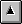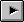## A.12 The optional Floating-Point word set

The Technical Committee has considered many proposals dealing with the inclusion and makeup of the Floating-Point Word Sets in ANS Forth. Although it has been argued that ANS Forth should not address floating-point arithmetic and numerous Forth applications do not need floating-point, there are a growing number of important Forth applications from spreadsheets to scientific computations that require the use of floating-point arithmetic. Initially the Technical Committee adopted proposals that made the Forth Vendors Group Floating-Point Standard, first published in 1984, the framework for inclusion of Floating-Point in ANS Forth. There is substantial common practice and experience with the Forth Vendors Group Floating-Point Standard. Subsequently the Technical Committee adopted proposals that placed the basic floating-point arithmetic, stack and support words in the Floating-Point word set and the floating-point transcendental functions in the Floating-Point Extensions word set. The Technical Committee also adopted proposals that:
• changed names for clarity and consistency; e.g., REALS to FLOATS, and REAL+ to FLOAT+ .
• removed words; e.g., FPICK .
• added words for completeness and increased functionality; e.g., FSINCOS, F~, DF@, DF!, SF@ and SF!

Several issues concerning the Floating-Point word set were resolved by consensus in the Technical Committee:

Floating-point stack: By default the floating-point stack is separate from the data and return stacks; however, an implementation may keep floating-point numbers on the data stack. A program can determine whether floating-point numbers are kept on the data stack by passing the string FLOATING-STACK to ENVIRONMENT? It is the experience of several members of the Technical Committee that with proper coding practices it is possible to write floating-point code that will run identically on systems with a separate floating-point stack and with floating-point numbers kept on the data stack.

Floating-point input: The current base must be DECIMAL. Floating-point input is not allowed in an arbitrary base. All floating-point numbers to be interpreted by an ANS Forth system must contain the exponent indicator E (see 12.3.7 Text interpreter input number conversion). Consensus in the Technical Committee deemed this form of floating-point input to be in more common use than the alternative that would have a floating-point input mode that would allow numbers with embedded decimal points to be treated as floating-point numbers.

Floating-point representation: Although the format and precision of the significand and the format and range of the exponent of a floating-point number are implementation defined in ANS Forth, the Floating-Point Extensions word set contains the words DF@, SF@, DF!, and SF! for fetching and storing double- and single-precision IEEE floating-point-format numbers to memory. The IEEE floating-point format is commonly used by numeric math co-processors and for exchange of floating-point data between programs and systems.

In defining custom floating-point data structures, be aware that CREATE doesn't necessarily leave the data space pointer aligned for various floating-point data types. Programs may comply with the requirement for the various kinds of floating-point alignment by specifying the appropriate alignment both at compile-time and execution time. For example:
```: FCONSTANT ( F:  r -- )
CREATE FALIGN  HERE  1 FLOATS ALLOT  F!
DOES> ( F:  -- r )  FALIGNED F@ ;
```

#### A.12.3.7 Text interpreter input number conversion

The Technical Committee has more than once received the suggestion that the text interpreter in Standard Forth systems should treat numbers that have an embedded decimal point, but no exponent, as floating-point numbers rather than double cell numbers. This suggestion, although it has merit, has always been voted down because it would break too much existing code; many existing implementations put the full digit string on the stack as a double number and use other means to inform the application of the location of the decimal point.

``` See: RFI 0004 Number Conversion ```

### A.12.6 Glossary

A.12.6.1.0558 >FLOAT

>FLOAT enables programs to read floating-point data in legible ASCII format. It accepts a much broader syntax than does the text interpreter since the latter defines rules for composing source programs whereas >FLOAT defines rules for accepting data. >FLOAT is defined as broadly as is feasible to permit input of data from ANS Forth systems as well as other widely used standard programming environments.

This is a synthesis of common FORTRAN practice. Embedded spaces are explicitly forbidden in much scientific usage, as are other field separators such as comma or slash.

While >FLOAT is not required to treat a string of blanks as zero, this behavior is strongly encouraged, since a future version of ANS Forth may include such a requirement.

A.12.6.1.1427 F.

For example, 1E3 F. displays 1000. .

A.12.6.1.1492 FCONSTANT

Typical use: `r FCONSTANT name`

A.12.6.1.1552 FLITERAL

Typical use: `: X ... [ ... ( r ) ] FLITERAL ... ;`

A.12.6.1.1630 FVARIABLE

Typical use: `FVARIABLE name`

A.12.6.1.2143 REPRESENT

This word provides a primitive for floating-point display. Some floating-point formats, including those specified by IEEE-754, allow representations of numbers outside of an implementation-defined range. These include plus and minus infinities, denormalized numbers, and others. In these cases we expect that REPRESENT will usually be implemented to return appropriate character strings, such as +infinity or nan, possibly truncated.

A.12.6.2.1489 FATAN2

FSINCOS and FATAN2 are a complementary pair of operators which convert angles to 2-vectors and vice-versa. They are essential to most geometric and physical applications since they correctly and unambiguously handle this conversion in all cases except null vectors, even when the tangent of the angle would be infinite.

FSINCOS returns a Cartesian unit vector in the direction of the given angle, measured counter-clockwise from the positive X-axis. The order of results on the stack, namely y underneath x, permits the 2-vector data type to be additionally viewed and used as a ratio approximating the tangent of the angle. Thus the phrase FSINCOS F/ is functionally equivalent to FTAN, but is useful over only a limited and discontinuous range of angles, whereas FSINCOS and FATAN2 are useful for all angles. This ordering has been found convenient for nearly two decades, and has the added benefit of being easy to remember. A corollary to this observation is that vectors in general should appear on the stack in this order.

The argument order for FATAN2 is the same, converting a vector in the conventional representation to a scalar angle. Thus, for all angles, FSINCOS FATAN2 is an identity within the accuracy of the arithmetic and the argument range of FSINCOS. Note that while FSINCOS always returns a valid unit vector, FATAN2 will accept any non-null vector. An ambiguous condition exists if the vector argument to FATAN2 has zero magnitude.

A.12.6.2.1516 FEXPM1

This function allows accurate computation when its arguments are close to zero, and provides a useful base for the standard exponential functions. Hyperbolic functions such as cosh(x) can be efficiently and accurately implemented by using FEXPM1; accuracy is lost in this function for small values of x if the word FEXP is used.

An important application of this word is in finance; say a loan is repaid at 15% per year; what is the daily rate? On a computer with single precision (six decimal digit) accuracy:

1. Using FLN and FEXP:

FLN of 1.15 = 0.139762, divide by 365 = 3.82910E-4, form the exponent using FEXP = 1.00038, and subtract one (1) and convert to percentage = 0.038%.

Thus we only have two digit accuracy.

2. Using FLNP1 and FEXPM1:

FLNP1 of 0.15 = 0.139762, (this is the same value as in the first example, although with the argument closer to zero it may not be so) divide by 365 = 3.82910E-4, form the exponent and subtract one (1) using FEXPM1 = 3.82983E-4, and convert to percentage = 0.0382983%.

This is full six digit accuracy.

The presence of this word allows the hyperbolic functions to be computed with usable accuracy. For example, the hyperbolic sine can be defined as:

```: FSINH  ( r1 -- r2 )
FEXPM1  FDUP  FDUP 1.0E0 F+  F/  F+  2.0E0 F/ ;
```

A.12.6.2.1554 FLNP1

This function allows accurate compilation when its arguments are close to zero, and provides a useful base for the standard logarithmic functions. For example, FLN can be implemented as:

```	: FLN   1.0E0 F-  FLNP1 ;
```

``` See: A.12.6.2.1516 FEXPM1 ```

A.12.6.2.1616 FSINCOS

``` See: A.12.6.2.1489 FATAN2 ```

A.12.6.2.1640 F~

This provides the three types of floating point equality in common use -- close in absolute terms, exact equality as represented, and relatively close.Table of ContentsNext Section